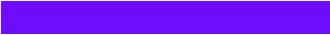MOVE CURSOR OVER ANSWERS: DO NOT CLICK MOUSE The Interactive technology of this page doesn't work with all tablets and mobile devices. If that is the case, Scroll down to bottom of page to check your answers.
 1. What does RER (respiratory exchange ratio) stand for?
 A. Metabolic equivalents in carbohydrate metabolism B. A fatigue ratio C. Ratio: VCO2/VO2 D. Reference measure of acidosis2. A VO2max test not meeting criteria is referred to as which of the following?
 A. VO2 subtrial B. VO2 peak C. VO2 subtest D. VO2 stress3. The average increase in VO2max, with untrained persons, from training studies is:
 A. 15% to 20% B. 20% to 30% C. 5% to 10% D. 10% to 15%4. What role does myoglobin play in aerobic exercise?
 A. Transport of oxygen in the blood B. Binds to electrons in the electron transport chain C. It shuttles the oxygen molecules from the cell membrane to mitochondria D. Shuttles hydrogens into the TCA cycle5. If you utilize fat more efficiently, how will this effect glycogen depletion?
 A. Spare glycogen B. No effect C. Increase glygogen usage D. None of the above6. What does ejection fraction mean?
 A. The volume of blood left after systole B. The percent of blood pumped out of the ventricle C. The volume of blood pumped per beat D. The volume of blood pumped per minute7. Which energy system predominates in a VO2max test?
 A. Phosphagen B. Glycolysis C. Mitchondrial respiration D. They all equally contribute to VO2max8. What would an average ejection fraction be at rest?
 A. 40% B. 50% C. 60% D. 70%9. SV increases with exercise until what % VO2 max (range) in untrained individuals?
 A. 70-90% B. 90-100% C.10-30% D. 40-60%10. How much blood flow is shifted to working muscle at maximal exercise?
 A. 55% to 60% B. 65% to 7-% C. 75% to 80% D. 85% to 90%11. The oxidation of fat means?
 A. An increase in lipid storage B. The breakdown or burning of fat C. Increase in fatty acids D. Decrease in mitochondria function12. During exercise, with a healthy person, what happens to diastolic blood pressure?
 A. It rises very similar to systolic blood pressure B. It rises more than systolic blood pressure C. It stays pretty level and may slightly lower D. None of the above13. Arteriole venous oxygen difference (a-VO2) refers to what?
 A. Increased hematocrit from increased plasma volume B. Redistribution of peripheral blood flow C. Peripheral blood flow D. Extraction of oxygen from the capillary bed14. Which is more associated to exercise intensity: HR or O2?A. Heart rate (HR) B. Oxygen consumption (O2) C. Varies with fitness level D. No difference
 15. How much does capillary supply increase (in %) due to aerobic training?
 A. >15% B. >20% C. >25% D. >30%16. Ideally a VO2 max protocol should last how long?
 A. 6 to 10 minutes B. 8 to 12 minutes C. 10 to 14 minutes D. 10 to 16 minutes17. With aerobic training, how much increase in oxidative enzymes is shown?
 A. 4.5 times (or 450%) B. 5 times (or 500%) C. 2.5 times (or 250%) D.3.5 times (or 350%)18. What is the mitochondrial density increase from aerobic exercise?
 A. 35% increase in number and 15% increase in size B. 15% increase in size and 25% increase in number C. 5% increase in size and 35% increase in number D. 15% increase in number and 35% increase in size Class, Stay Laser Focused with your Studying!19. The atrial contraction sends what %of blood to the ventricles?A. 10% B. 20% C. 30% D. 40%
 20. Cardiac output is equal to?
 A. Stroke volume times heart rate B. ESV/EDV C. ESV x EDV D. Ejection fraction X 10021. Which Ventricular volume will always be the largest value?
 A. SV B. EDV C. ESV D. It varies with all people22. What are the theoretical limits of the respiratory exchange ratio?
 A. .4 to 1.0 B. .5 to 1.0 C. .6 to 1.0 D. ,7 to 1.023. What does buffering capacity refer to?
 A. Enzyme activity in the muscle B. Speed of hydrolysis of ATP C. The muscle’s capacity to neutralize the acid that accumulates during glycolysis D. Dynamic muscle action‘s contraction capability24. When asked, 'what is the optimal intensity for a fat burning workout', which of the following answers is the best recommendation?
 A. Exercise where you feel the burn B. To burn more fat, burn more calories (comfortable but challenging intensity) C. Choose a catabolic intensity D. Train at 70% to 80% of your maximum heart rate25. Mathematically, which of the following represents the FICK equation?
 A. VO2 = Q x a-v O2 difference B. VO2 = SV x HR x a-v O2 difference C. VO2 = (EDV - ESV) x HR x a-v O2 difference D. All of the aboveComplete!
 1=C 2=B 3=A 4=C 5=A 6=B 7=C 8=C 9=D 10=D 11=B 12=C 13=D 14=B 15=A 16=B 17=C 18=D 19=C 20=A 21=B 22=D 23=C 24=B 25=ALLTop of Page | Research Interests | Vita | Articles | New Projects | Miscellaneous | UNM | Home